# 从闭包和高阶函数初探JS设计模式

JavaScript是一门完整的面向对象的编程语言，JavaScript在设计之初参考并引入了Lambda表达式、闭包和高阶函数等特性。

# 一、闭包（Closure）

## 1.1 变量的作用域

``````var a = 0;
function func() {
var a = 1;
var b = 2;
console.log(a, b);
}
func();          // output: 1 2;
console.log(a);  // output: 0
console.log(b);  // output: Uncaught ReferenceError: b is not defined

``````var a = 1;
var func = function() {
var b = 2;
var func2 = function() {
var c = 3;
console.log(a);
console.log(b);
console.log(c);
}
func2();
console.log(c);
}
func();

``````1
2
3
Uncaught ReferenceError: c is not defined

## 1.2 变量的生命周期

``````function func() {
var a = 1;      //函数执行完成后将自动销毁
console.log(a)
}
func();

## 1.3 闭包改变局部变量的生命周期

``````function func() {
var a = 0;      //函数执行完成后将自动销毁
return function() {
a = a + 1;
console.log(a);
}
}
var f = func();

f();    // output: 1
f();    // output: 2
f();    // output: 3
f();    // output: 4
console.log(a);  // output: Uncaught ReferenceError: a is not defined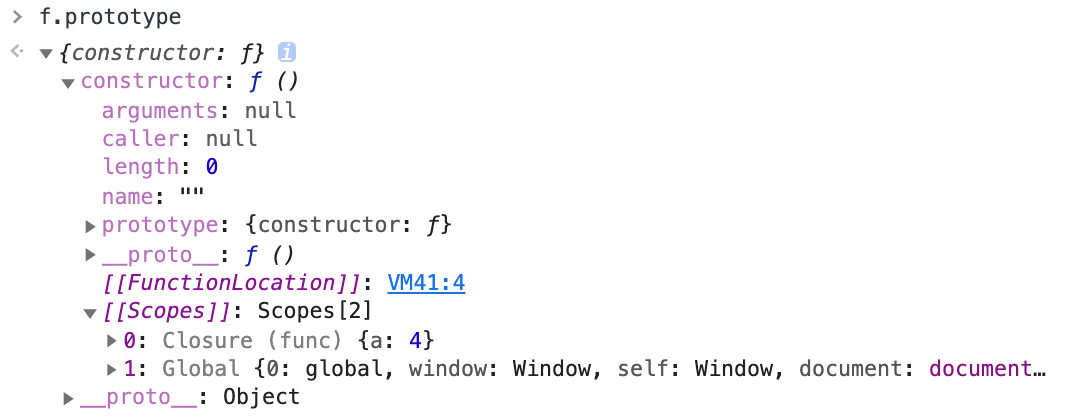## 1.4 闭包的更多作用

“闭包”可以改变局部变量的生命周期，并且不更改局部变量的作用范围，这一特性使得闭包的运用非常广泛。

### 1.4.1 缓存

``````const multiplication = (function() {
const cache = {};
return function() {
const args = Array.prototype.join.call(arguments, ',');
if (args in cache) {
return cache[args];
}
let sum = 1;
for (let i = 0; i < arguments.length; i++) {
sum = sum * arguments[i];
}
return cache[args] = sum;
}
})();

multiplication(1,2,3,4);

``````const multiplication = (function() {
const cache = {};

const calculate = function() {
let sum = 1;
for (let i = 0; i < arguments.length; i++) {
sum = sum * arguments[i];
}
return sum;
}

return function() {
const args = Array.prototype.join.call(arguments, ',');
if (args in cache) {
return cache[args];
}
return cache[args] = calculate.apply(null, arguments);
}
})();

multiplication(1,2,3,4);

### 1.4.2 面向对象编程：

``````/****************** 写法1 *******************/
var Person = function() {
var age = 18;
return {
age++;
console.log('age:', age);
}
}
}
var person = Person();

/****************** 写法2 *******************/
var person = {
age: 18,
this.age = this.age + 1;
console.log('age:', this.age);
}
};

/****************** 写法3 *******************/
var Person = function() {
this.age = 18;
}
this.age++;
console.log(this.age);
}
var person = new Person();

# 二、高阶函数（HOF）

1. 函数可以作为参数被传递
2. 函数可以作为返回值输出

## 2.1 简单示例

``````const getSingleBuider = function(fn) {
let instance;
return function() {
return instance || (instance = fn.apply(this, arguments));
}
}

## 2.2 高阶函数与AOP

AOP（面向切面编程）的主要作用是把一些跟核心业务逻辑无关的功能抽离出来，例如日志统计、异常处理、安全控制等。将这些功能抽离后，再通过“动态织入”的方式掺入业务逻辑模块中。能够保证业务逻辑模块的高内聚，以及抽离的功能能够很好的复用。

``````Function.prototype.before = function(beforeFn) {
var _self = this;                           // 存储原函数的引用
// 返回原函数与新函数的“代理”函数
return function() {
beforeFn.apply(this, arguments);        // 执行新函数
return _self.apply(this, arguments);    // 执行原函数返回执行结果
}
}
Function.prototype.after = function(afterFn) {
var _self = this;
return function() {
const result = _self.apply(this, arguments);
afterFn.apply(this, arguments);
return result;
}
}

let func = function() {
console.log('run');
}

func = func.before(function(){
console.log('berfore run');
}).after(function() {
console.log('after run');
});

func();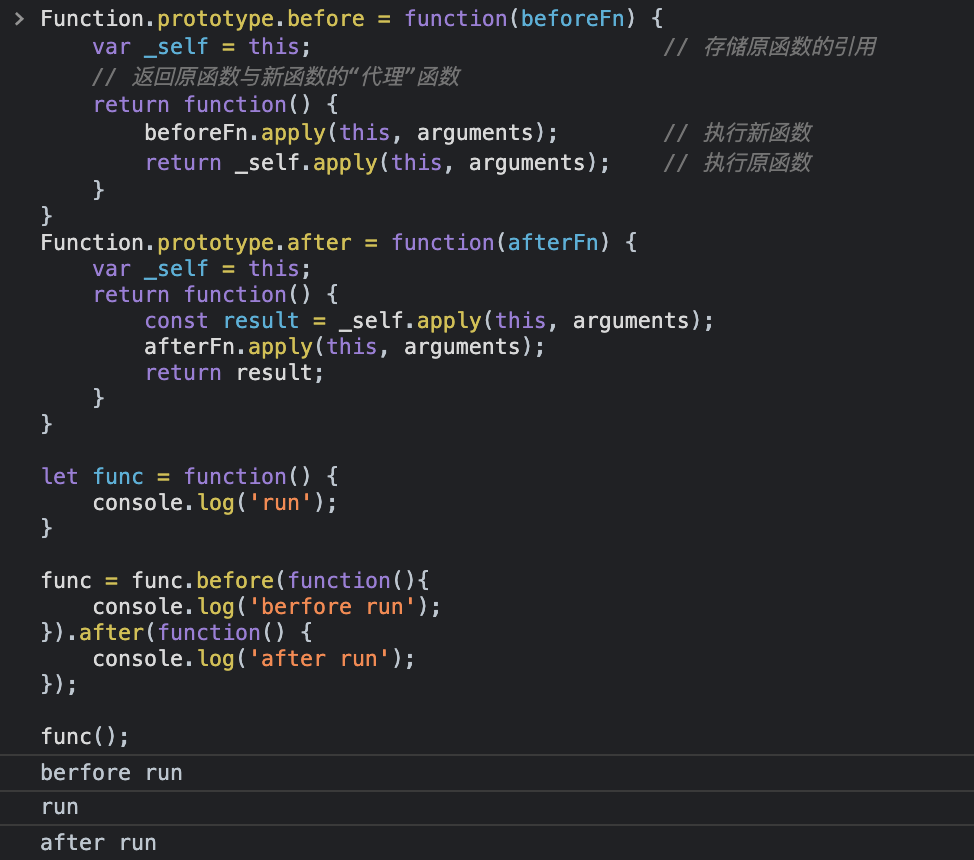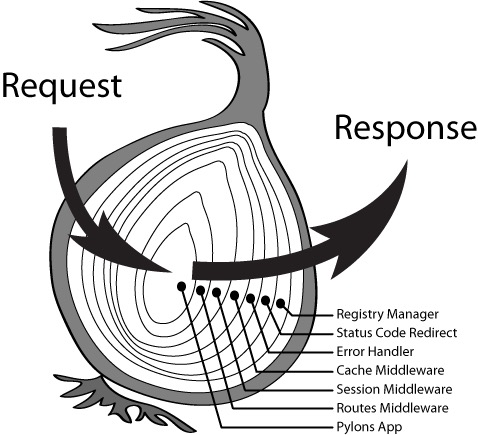## 2.3 柯里化（Curring）

``````sum(1)(2)(3); // output: 6

``````function sum(a) {
return function(b) {
return function(c) {
return a + b + c;
}
}
}
sum(1)(2)(3);  // output: 6

``````// 柯里化函数第一版
function curry(fn) {
// 将传入的函数fn从实参数组中移除
const args = Array.prototype.slice.call(arguments, 1);
// 返回函数用于接受下一个参数
return function() {
// 将返回函数需要接受的下一次入参保存到newArgs中，slice浅拷贝
const newArgs = args.concat(Array.prototype.slice.call(arguments));
// 将newArgs参数放到被柯里化函数中执行
return fn.apply(this, newArgs);
};
}

return a + b;
}

// 或者
// 或者

``````const curry = function(fn) {
return function inner() {
// 浅拷贝入参
const args = Array.prototype.slice.call(arguments);
// 如果下一个参数的长度大于了函数的行参个数，则跳出递归
if (arguments.length >= fn.length) {
return fn.apply(undefined, args);
} else {
// 否则继续处理后续参数，返回curring函数
return function() {
// 获取合并上一次和下一次的入参
const allArgs = args.concat(Array.prototype.slice.call(arguments));
return inner.apply(undefined, allArgs);
};
}
};
}

function sum(a, b, c) {
return a + b + c;
}

const currySum = curry(sum);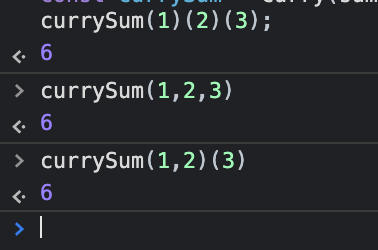``````const curry = fn =>
judge = (...args) =>
args.length >= fn.length
? fn(...args)
: arg => judge(...args, arg);## 2.5 分时函数

``````<!DOCTYPE html>
<html lang="en">
<meta charset="UTF-8">
<meta http-equiv="X-UA-Compatible" content="IE=edge">
<meta name="viewport" content="width=device-width, initial-scale=1.0">
<title>分时函数</title>
<body>
<div>分时函数性能优化验证</div>

<script>
// 一次性添加到页面
const dataSource = new Array(10000).fill('DYBOY');
// 创建DOM
const createDiv = (text = 'DYBOY') => {
const div = document.createElement('div');
div.innerHTML = text;
document.body.appendChild(div);
}
// 批量添加
for (data of dataSource) {
createDiv(data);
}
</script>
</body>
</html>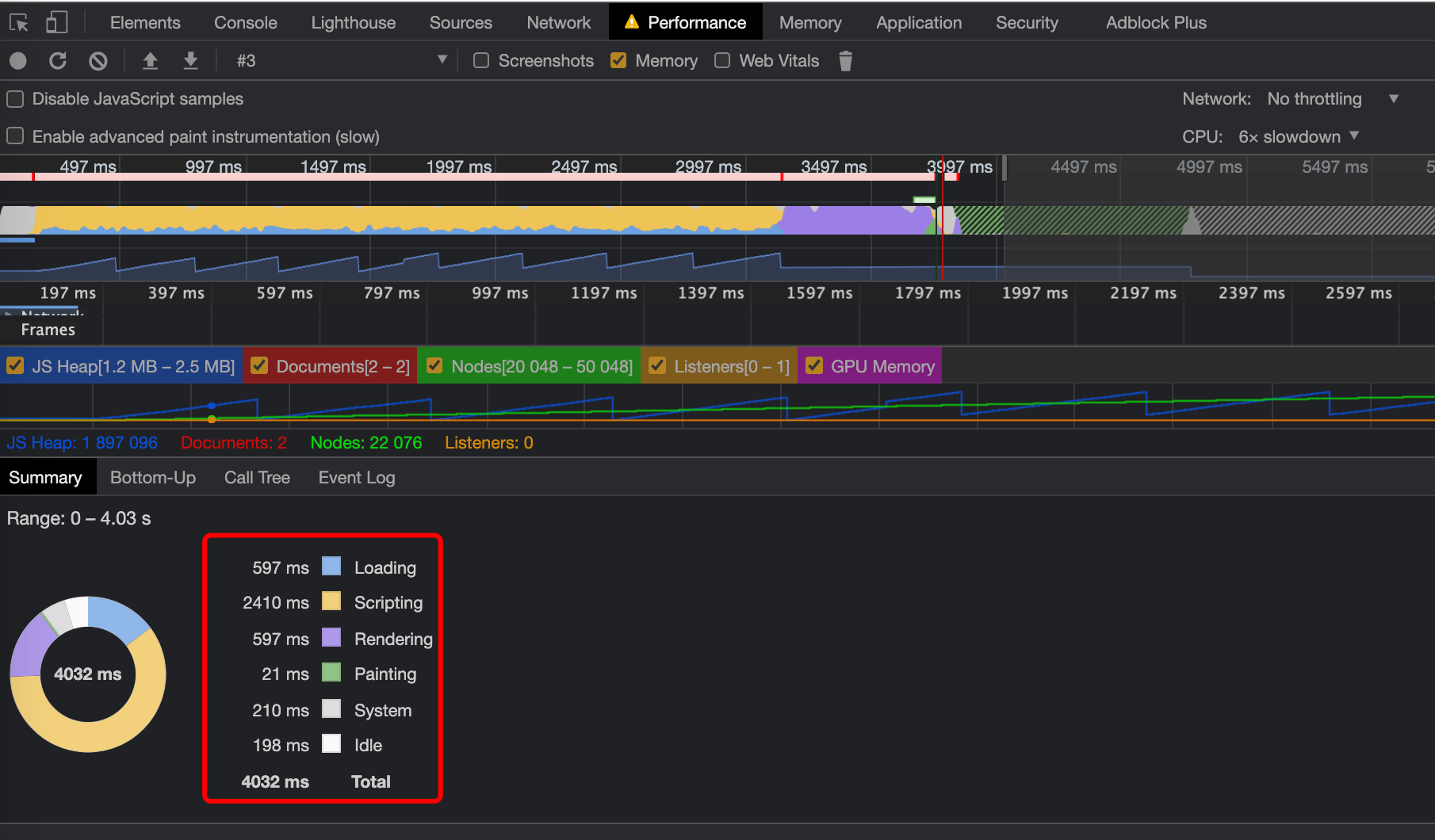``````<!DOCTYPE html>
<html lang="en">
<meta charset="UTF-8">
<meta http-equiv="X-UA-Compatible" content="IE=edge">
<meta name="viewport" content="width=device-width, initial-scale=1.0">
<title>分时函数</title>
<body>
<div>分时函数性能优化验证</div>

<script>
// 一次性添加到页面
const dataSource = new Array(10000).fill('DYBOY');
// 创建DOM
const createDiv = (text = 'DYBOY') => {
const div = document.createElement('div');
div.innerHTML = text;
document.body.appendChild(div);
}
// 批量添加
// for (data of dataSource) {
//     createDiv(data);
// }

/**
* 分时函数
* @param dataSource - 数据数组
* @param fn - 分时执行的函数
* @param count - 每分段时间内执行函数的次数
* @param duration - 分段时长，单位ms
**/
const timeChunk = (dataSource, fn, count = 1, duration = 200) => {
let timer;
const start = () => {
const minCount = Math.min(count, dataSource.length);
for(let i = 0; i < minCount; i++) fn(dataSource.shift());
}
return () => {
timer = setInterval(() => {
if (dataSource.length === 0) return clearInterval(timer);
start();
}, duration);
}
}

const newRender = timeChunk(dataSource, createDiv, 100, 300);

newRender();
</script>
</body>
</html>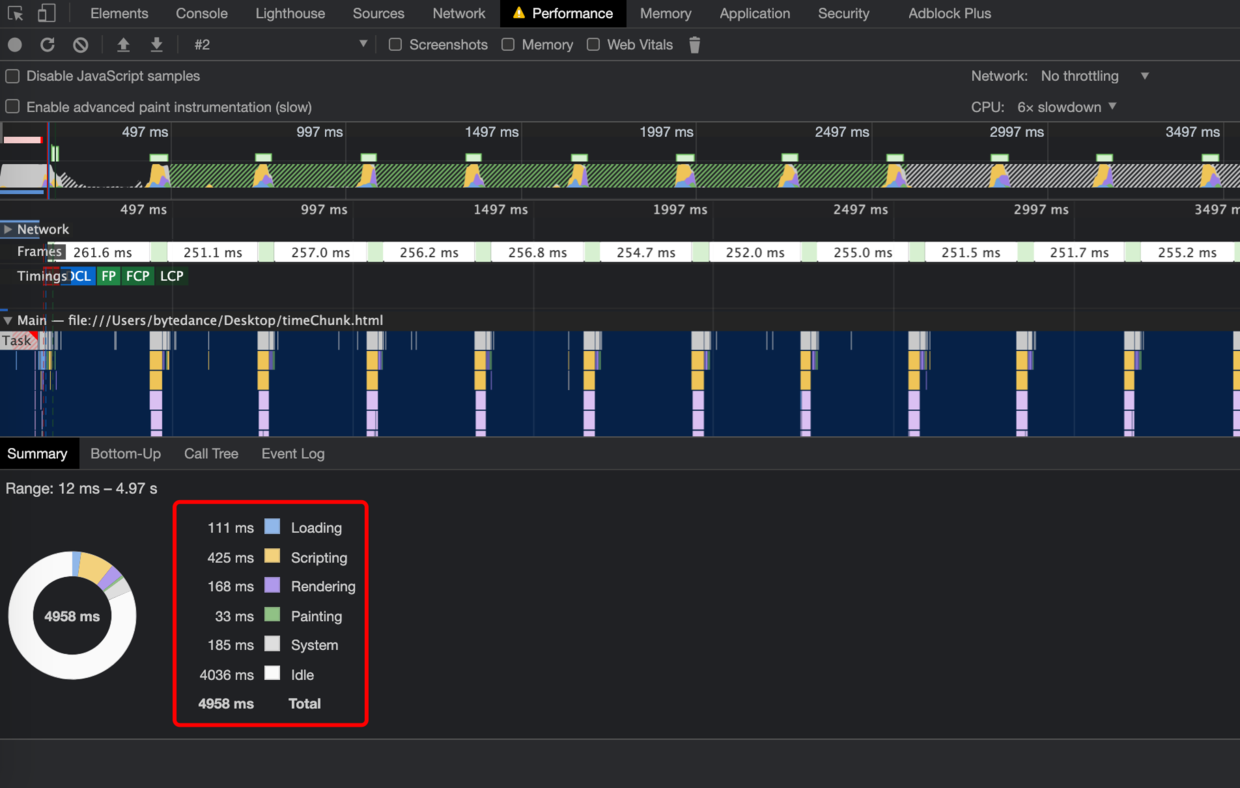## 2.6 惰性加载函数

1. 在函数调用时处理：函数内部复写函数，直接返回值；
2. 在函数声明时处理：函数声明时，确定返回值。